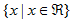Domain

The domain of a function is the set of values of the independent variable (x) for which the function is defined.

There are two common mathematical operations that are undefined: square root of a negative number and division by zero. Since linear functions do not include either of these operations, their domain is usually all real numbers.

If the value of x is not restricted by the situation the function is modeling, then the domain can be defined as all real numbers. This is written. The domain will provide the x coordinates for the function graph.